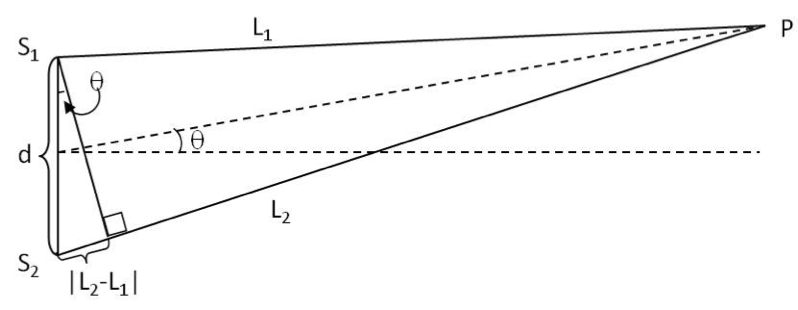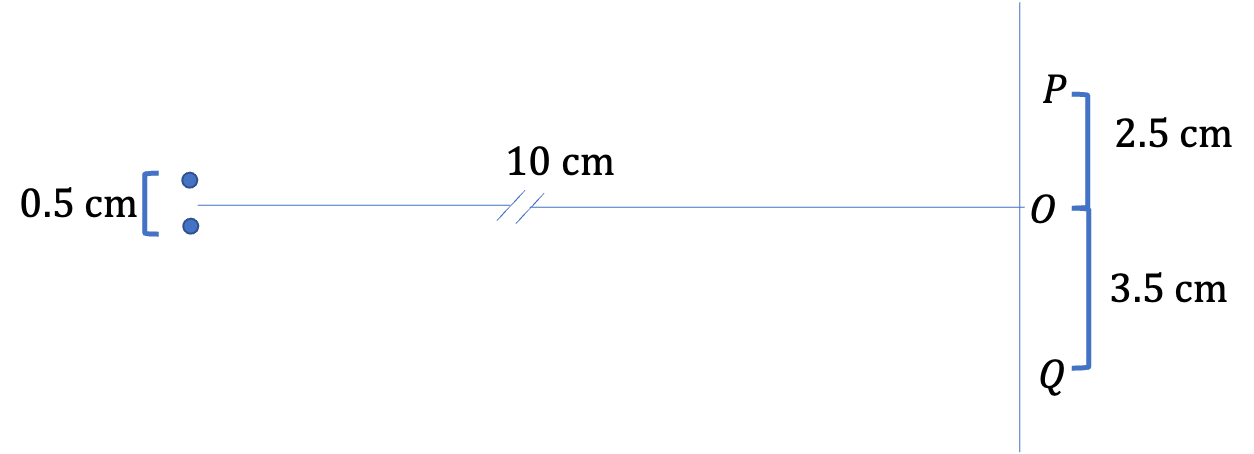## Section14.9Interference of Waves

When a wave results from superposition of two waves with diffenent paths, their net intensity may have unexpected results. The resulting pattern is called interference pattern and the phenomenon is referred to as interference.

Let $\psi_1$ and $\psi_2$ be two waves overlapping in a particular region of space. By superposition principle, the resultant wave would be the sum of $\psi_1$ and $\psi_2\text{.}$

\begin{equation*} \psi = \psi_1 + \psi_2. \end{equation*}

This will happen at each point and at each instant. I will denote position by $\vec r$ and time by $t\text{.}$ Therefore, with these details, this equation will look like

\begin{equation} \psi(\vec r, t) = \psi_1(\vec r, t) + \psi_2(\vec r, t).\tag{14.9.1} \end{equation}

We are interested in average power at position $\vec r\text{.}$ Here average means time-averaging the square of the wave.

\begin{equation} P_\text{av} \propto \left( \psi^2 \right)_\text{time average}. \tag{14.9.2} \end{equation}

Let us denote time averaging by enclosing the quantity to be time averaged by angle brackets $\langle \rangle$ so that we write less. This gives intensity of the wave to be

\begin{equation} I \propto \langle \psi^2 \rangle = \langle (\psi_1 + \psi_2)^2 \rangle.\tag{14.9.3} \end{equation}

Expanding the square inside time averaging we get

\begin{equation} I \propto \langle \psi_1^2 \rangle + \langle \psi_2^2 \rangle + \langle 2\psi_1\psi_2 \rangle.\tag{14.9.4} \end{equation}

Therefore, we will get

\begin{equation} I = I_1 + I_2 + \langle 2\psi_1\psi_2 \rangle,\tag{14.9.5} \end{equation}

where $I_1$ is the intensity from wave $\psi_1$ alone and $I_2$ the intensity from wave $\psi_2$ alone. The reamining term, $\langle 2\psi_1\psi_2 \rangle$ is the new effect. Its called the interference term, usually denoted by $I_{12}\text{.}$ Clearly,

\begin{equation} I \ne I_1 + I_2. \tag{14.9.6} \end{equation}

That is the surprise effect. While the intensities of the two waves $I_1$ and $I_2$ are positive numbers, the interference term $I_{12}$ can be positive or negative. Therefore the actual intensity observed can be different than simply the sum of the intensities of the separate waves. As a matter of fact, if the two waves have equal intensities to begin with, then the net wave can have intensity anywhere between zero and four times the intensity of one of the waves, not just twice.

### Subsection14.9.1Mixing Two Waves of Equal Amplitude

For a simple example, consider two waves of equal frequency and equal amplitude. Let the two waves start out at $S_1$ and $S_2$ in synchronization with each other, but wave 1 travels a distance $L_1$ to reach a point P at the detector while wave 2 travels a distance $L_2$ to reach the same point as shown in the Figure 14.9.1.

This experiment can be set up, for example, by having two slits in a plate through which waves must exit on the other side and move to $P$ where they must produce a net vibration. For instance, we can shine light from a laser on an opaque plate with two slits at $S_1$ and $S_2\text{.}$ The experimental arrangement with two slits is also called Young's double slit experiment who studied interference of light waves by this method.

Even if the two waves start out in sync at $S_1$ and $S_2\text{,}$ they might not be in sync at point $P$ on the screen, depending upon relative distances from the source points to $P\text{.}$ If the difference between $L_1$ and $L_2$ is a multiple of a wavelength, then we expect them to end up in sync again at $P$ and a maximum intensity should result there. When this happens, we say that the two waves have a constructive interference at point $P\text{.}$ This is shown in Figure 14.9.2 where two waves which start out at points $S_1$ and $S_2$ in sync end up in sync at point $P\text{.}$

On the other hand, if the difference of distances from the sources at $S_1$ and $S_2$ to the point $P$ is an odd multiple of half a wavelength, then the crest of one wave will fall on the trough of the other, making the net amplitude zero there. The waves are said to interfere destructively at point $P\text{.}$ If the amplitudes of the waves from $S_1$ and $S_2$ are equal, then, the net amplitude at the point of destructive interference will be zero since the two waves will be completely out of sync there. This is shown in Figure 14.9.3 where two waves which start out in sync at points $S_1$ and $S_2$ end up out of sync at point $P$ and interfere destructively there.

Let us now see how the constructive and destructive interferences show up analytically as a result of the addition of the amplitudes of the two waves. For this, we need to work out the interference term more fully.

\begin{equation*} I_{12} = 2 \alpha \langle \psi_1 \psi_2 \rangle. \end{equation*}

Since waves 1 and 2 travel distances $L_1$ and $L_2$ their wave functions at point $P$ will take on the following values at an arbitrary time $t\text{.}$

\begin{align*} \amp \psi_1(t) = A \cos\left(kL_1 - \omega t \right) \\ \amp \psi_2(t) = A \cos\left(kL_2 - \omega t \right) \end{align*}

Note the waves have the same frequency, wavenumber and amplitude for our exercise here. Note also that we have dropped the phase constant since we have assumed that the waves start out in sync at $S_1$ and $S_2\text{.}$ Multiplying the two wave functions we find

\begin{align*} \psi_1(t)\psi_2(t) \amp = A^2 \cos\left(kL_1\right)\cos\left(kL_2\right) \cos^2\omega t \\ \amp \ \ \ + A^2 \sin\left(kL_1\right)\sin\left(kL_2\right) \sin^2\omega t\\ \amp \ \ \ - A^2 \cos\left(kL_1\right)\sin\left(kL_2\right) \cos\omega t \sin\omega t\\ \amp \ \ \ - A^2 \sin\left(kL_1\right) \cos\left(kL_2\right) \cos\omega t \sin\omega t \end{align*}

We need to find time-average of this. That involved calculating time averages, $\langle \cos^2\omega t rangle\text{,}$ $\langle \sin^2\omega t rangle\text{,}$ and $\langle \cos\omega t \sin\omega t rangle\text{.}$ The time average of a periodic function can be easily done by an integration over one period and dividing by the period. Let $T$ be the period.

\begin{equation} \langle f(t) \rangle = \frac{1}{T}\int_0^T f(t) dt. \tag{14.9.7} \end{equation}

By using this definition, we get

\begin{align} \amp \langle \cos^2(\omega t)\rangle = \frac{1}{T}\int_0^T \cos^2(\omega t) dt = \frac{1}{2}\tag{14.9.8}\\ \amp \langle\sin^2(\omega t)\rangle = \frac{1}{T}\int_0^T \sin^2(\omega t) dt = \frac{1}{2}\tag{14.9.9}\\ \amp \langle \cos(\omega t)\sin(\omega t)\rangle = \frac{1}{T}\int_0^T \cos(\omega t)\sin(\omega t) dt = 0\tag{14.9.10} \end{align}

Therefore, the interference term evaluates to

\begin{equation*} I_{12} =\alpha A^2 \cos\left[k\left( L_1 -L_2 \right)\right]. \end{equation*}

Note the intensity of each wave is

\begin{equation*} I_{1}= I_{2} \propto \langle \psi_1^2\rangle = \frac{1}{2}\alpha A^2. \end{equation*}

Let us write the intensity of the individual waves as $I_0\text{.}$

\begin{equation*} I_0 \equiv I_1 = I_2. \end{equation*}

Now, writing the intensity observed at point P, all in terms of $I_0\text{,}$ the intensity of separate waves, is

\begin{equation} I = 2I_0\left\{ 1+ \cos\left[k\left( L_1 -L_2 \right)\right]\right\}\label{eq-intensity-as-difference-lengths}\tag{14.9.11} \end{equation}

For fixed source points $S_1$ and $S_2\text{,}$ the intensity depends upon the location of the observation point $P$ since depending upon the value of $k(L_1-L_2)$ the cosine function fluctuates between $-1$ and $1\text{.}$ When cosine is equal to $-1\text{,}$ the intensity at $P$ will be zero, and when cosine is $+1\text{,}$ the intensity is four times as much as the intensity in each wave. Hence, we have the following conditions for constructive and destructive interference.

\begin{equation*} k(L_1 - L_2) = \begin{cases} 2n\pi \amp n = 0, \pm 1, \pm 2, \cdots \text{Constructive} \\ (2n+1)\pi \amp n = 0, \pm 1, \pm 2, \cdots \text{Destructive} \end{cases} \end{equation*}

Let us write the wave number k in terms of the wavelength by using $k = 2\pi/\lambda\text{,}$ we get a simpler form that clearly shows the path difference versus integral and half-integral multiples of wavelength.

\begin{equation*} L_1 - L_2 = \begin{cases} n\lambda \amp n = 0, \pm 1, \pm 2, \cdots \text{Constructive} \\ \left( n + \frac{1}{2}\right)\lambda \amp n = 0, \pm 1, \pm 2, \cdots \text{Destructive} \end{cases} \end{equation*}

Therefore, the waves from two synchronized sources of the same frequency interfere constructively when the distances to an observation point $P$ differ by multiples of a wavelength and interfere destructively if the distances differ by half a wavelength, or odd multiples of half a wave length.

### Subsection14.9.2Simplification for Large $L$

Often we are interested in interference of two waves far away from the sources. In this case, we can use trigonometry to simplify the interference conditions when the difference in the path lengths of the two sources to the detector is small compared to the distance to the detector.

From Figure 14.9.4, we can find that the difference in paths is related to the angle $\theta$ and the separation $d$ of the sources.

\begin{equation*} \left| L_1-L_2 \right| = d\sin\theta \end{equation*}Figure 14.9.4. Geometry for approximating the difference in the path length from the source points and a point on the screen.

Therefore, the conditions for interference are:

\begin{equation*} d\sin\theta = \begin{cases} n\lambda \amp n = 0, \pm 1, \pm 2, \cdots \text{Constructive} \\ \left( n + \frac{1}{2}\right)\lambda \amp n = 0, \pm 1, \pm 2, \cdots \text{Destructive} \end{cases} \end{equation*}

Using $\left| L_1-L_2 \right| = d\sin\theta\text{,}$ we can express intensity from Eq. (14.9.11) in tems of direction from the slits and slit width as follows. I also rreplace $k$ by $2\pi/\lambda$ for ease of use.

\begin{equation} I = 2I_0\left[ 1+ \cos \left( \frac{2\pi d}{\lambda} \sin\theta \right) \right].\label{eq-intensity-in-direction-theta}\tag{14.9.12} \end{equation}

Waves of wavelength $50\text{ cm}$ are emitted from two point sources separated by $70\text{ cm}\text{.}$ In what directions will there be constructive interferences at a far away detector?

Hint

Use the formulas for large $L\text{.}$

Only $n = 0, \pm 1$ giving $\theta=0, \pm 45.6^{\circ}\text{.}$
Solution

This example illustrates the use of the formulas for constructive and destructive interferences in a double-slit experiment. We have been given the wavelength and the distance between the slits. Since the detector is far away, we can use the approximate formula for the interference conditions which gives the directions in which constructive and destructive interferences will occur in terms of the wavelength $\lambda$ and the distance $d$ between the slits. The constructive interferences are in the directions given by:

\begin{equation*} \sin\theta = \frac{n\lambda}{d},\ \ n = 0, \pm 1, \pm 2, \cdots. \end{equation*}

Clearly, the conditions fails for $n$ for which $\sin\theta>1$ since sine of an angle must be between -1 and 1, i.e. $-1\le\sin\theta\le1\text{.}$ Also, the allowed angles are symmetric about $\theta = 0$ since if $n=1$ is allowed, then $n=-1$ is also allowed. Now, we systematically work out the allowed angle starting with $n=0\text{.}$ We find the following allowed the directions of constructive interference:

\begin{align*} \amp n = 0:\ \ \theta=0\\ \amp n = \pm 1:\ \ \theta=\arcsin{\left( \frac{\pm 1 \times 50}{70}\right)} = \pm 45.6^{\circ}\\ \amp n = \pm 2:\ \ \theta=\arcsin{\left( \frac{\pm 2 \times 50}{70}\right)}\ \ \Longrightarrow \text{no solution} \end{align*}

Hence, only three constructive places are possible for the given conditions.

Two coherent $3\text{-mm}$ wavelength sources separated by $5\text{-mm}$ shine towards a screen as shown. If the intensity of individual source at the screen is $20\text{ W/m}^2\text{,}$ what is the intensity at points P and Q marked on the screen?Hint

Find angles to P and Q and use the intensity formula, Eq. (14.9.12).

$I_P = 39.3\text{ W/m}^2\text{,}$ $I_Q = 0.095\text{ W/m}^2\text{.}$
First we find angle $\theta$ to points P and Q. From the given geometry, we get
Now, we use these values in Eq. (14.9.12) to find intensity expected at those points. We need rto make sure we change the unit of wavelength to $\text{cm}$ so that all length units in the argument of cosine cancel out.